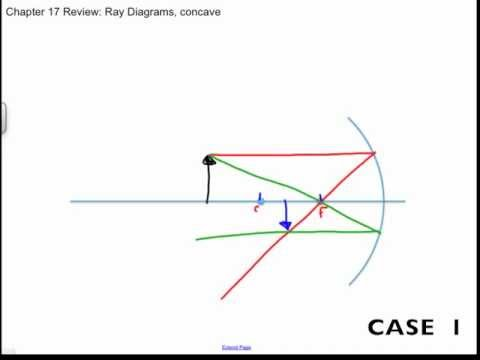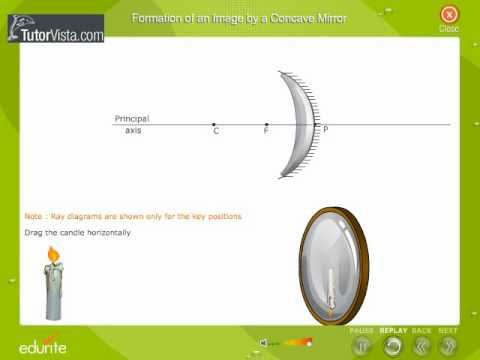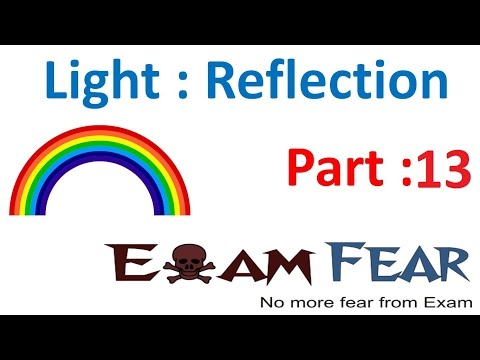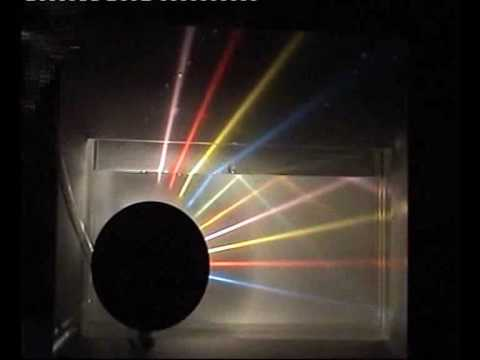## Mirror

Subject: Science

#### Overview

This note presents us the information about spherical mirrors, terms used in a spherical mirror, the position and the nature of the image formed by a concave mirror and differences between concave and convex mirror. Refraction with its laws is briefly described.
##### Mirror

A mirror is an object that reflects light in such a way that you can see your image in it whether real or virtual, erect or inverted and diminished or enlarged. Plane mirrors are the common mirrors used in our home. This is different from other light-reflecting objects that does not preserve much of the original wave signal other than colour and diffuse reflected light.

#### Types of mirror

There are two types of mirror they are:

1. plane mirror
2. spherical mirror

#### Plane mirrorA plane mirror is a mirror with a flat refractive surface. We use the plane mirror as a looking glass. Plane mirrors are the only type of mirrors for which a real object always produces an image that is virtual, erect and of the same size as the object.

#### Spherical mirror

A mirror whose polished, reflecting surface is a part of a hollow sphere of glass, is called a spherical mirror. One side of it is made opaque and another side acts as a reflecting surface.

Depending on the nature of the reflecting surface of the mirror, the mirrors are of two types:

#### 1. Concave mirrorA mirror which is a part of the sphere and is polished from the outer surface such as its reflecting side is inward is called a concave mirror. In this mirror, parallel rays of light converge at a point after reflection. A concave mirror is used to convert solar energy into heat energy, in torches and car's headlights to reflect the light of the bulb to form a powerful beam of light, in an astronomical telescope to view stars and planets.

Uses of Concave mirror

1. Concave mirrors are used as shaving glass.
2. Concave mirrors are used as reflectors in car headlights, searchlights, torches, etc.
3. Concave mirrors are used in projectors and telescopes.
4. Concave mirrors are used to observe the ear and nose of patients.

#### 2.Convex mirrorA mirror which is a part of the sphere and is silver polished from the inner surface such as its reflecting side is outward is called a convex mirror. In this mirror, parallel rays of light diverge after reflection. An image formed in the convex mirror is always virtual, erect and diminished. The convex mirror is used as rear view mirrors in cars, motorcycle, scooters, trucks, etc.

Uses of Convex mirror

1. The convex mirrors are used in cars that give the clear view of the vehicles approaching from behind.
2. It can diverge the light over a larger area and, therefore, it is used as a street light reflector.

Differences between Concave and Convex mirror

 Concave mirror Convex mirror A spherical mirror whose inner hollow surface is the reflecting surface is called a concave mirror. A spherical mirror whose outer bulging surface is the reflecting surface is called a convex mirror. It may give magnified or diminished image. It forms diminished image (smaller in size only). The image formed is real except when the object is between focus and principal. The image formed is always virtual.

Terms used in spherical mirror:

1. Pole: It is a geometrical centre of the surface of a mirror. It is represented by a point P.

2. Center of Curvature: It is the centre of the hollow sphere of which mirror is a part, represented by C.

3. The radius of Curvature: It is the radius of the hollow sphere of which mirror is a part, denoted by R.

4. Principal axis: The line joining the pole of the mirror and the centre of curvature and produced on both sides is called the principal axis.

5. Principal focus: The point on the principal axis where a beam of light incident to spherical mirror parallel to the principal axis converges or appears to be diverging from after reflection, is called the principal focus. It is denoted by the letter F. The principal focus is real in the concave mirror and virtual in the case of a convex mirror.

6. Focal length: It is the distance between principal focus and pole of the mirror. It is denoted by f. The focal length is half of the radius of curvature.

#### Rules Used to Draw Diagrams in Concave mirror:

1. An incident ray passing parallel to the principal axis on the way to the mirror will pass through the focal point upon reflection.2. An incident ray passing through the focal point on the way to the mirror will travel parallel to the principal axis upon reflection.3. An incident ray passing through the centre of curvature reflects back in the same direction.Ray diagram for Concave Mirror

1. If an object is at infinity from the mirror then the image of the mirror forms in the focal point.2. If an object is kept beyond the centre of curvature then image forms in between the centre of curvature and focus.#### Rules Used to Draw Diagrams in Convex mirror

1. An incident ray travelling parallel to the principal axis on the way to a convex mirror will reflect in such a manner that its extension will pass through the focal point.2. In the convex mirror, focal point and centre of curvature lie in the backside or reflecting the surface of a mirror.3. An incident ray passing through the centre of curvature reflects back in the same direction.Ray diagram for Convex Mirror

1. If an object is kept at infinity then the image of an object is formed at the focal point of the reflecting surface. Such images are virtual, erect and diminished.2. If an object is kept between the pole of mirror and infinity then the image is formed between the pole of the mirror and focal point. Such images are Virtual, erect and diminished.Sign convention for spherical mirrors

1. All distances are measured from the pole of the mirror.
2. Distances measured in the direction of the incident ray are positive.
3. Distances measured in the direction opposite to that of the incident rays are negative.
4. Distances measured below the principal axis are the negative i.e. height of a real inverted.
5. Distances measured from the principal axis are positive, i.e. heights of an erect image are positive.

##### Things to remember
• A mirror whose polished, reflecting surface is a part of hollow sphere of glass, is called a spherical mirror. One side of it is made opaque and another side acts as a reflecting surface.
• Concave mirror : A mirror which is a part of sphere and is polished from the outer surface such that its reflecting side is inward is called a concave mirror.
• Convex mirror : A mirror which is a part of sphere and is silver polished from the inner surface such that its reflecting side is outward is called a convex mirror.
• It includes every relationship which established among the people.
• There can be more than one community in a society. Community smaller than society.
• It is a network of social relationships which cannot see or touched.
• common interests and common objectives are not necessary for society.
##### Videos for Mirror##### Cbse Class 12 focal length Convex Mirror Physics Practical Experiment Video##### Concave and Convex Mirror Ray Diagrams, Chapter 17 Review##### Experiments on refraction, reflection and total internal reflection##### Formation Of An Image By a Concave Mirror##### Physics Light Reflection & Refraction part 13 (Refraction , Refractive index) CBSE class 10 X##### Questions and Answers

A mirror whose polished, reflecting surface is a part of a hollow sphere of glass is called a spherical mirror.

A spherical mirror whose outer surface is reflecting surface is called a convex mirror.

A spherical mirror whose inner surface is reflecting surface is called a convex mirror.

A concave mirror is also called converging mirror because a parallel beam of light incident on it, converges to a point after reflection.

Convex mirror is also called a diverging mirror because a parallel beam of light incident on it, appears to be diverging from a point after reflection.